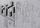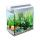Surface area + unit conversion - math problems

1. SphereThe surface of the sphere is 12100 cm2, and weight is 136 kg. What is its density?
2. PrismRight angle prism, whose base is right triangle with leg a = 7 cm and hypotenuse c = 10 cm has same volume as a cube with an edge length of 1 dm. a) Determine the height of the prism b) Calculate the surface of the prism c) What percentage of the cube
3. Tetrahedral pyramidCalculate the volume and surface area of a regular tetrahedral pyramid, its height is \$b cm and the length of the edges of the base is 6 cm.
4. Sphere slicesCalculate volume and surface of a sphere, if the radii of parallel cuts r1=31 cm, r2=92 cm and its distance v=25 cm.
5. Pyramid a+hCalculate the volume and surface area of the pyramid on the edge and height a = 26 cm. h = 3 dm.
6. CanopyMr Peter has metal roof cone shape with a height of 127 cm and radius 130 cm over well. He needs paint the roof with anticorrosion. How many kg of color must he buy if the manufacturer specifies the consumption of 1 kg to 3.3 m2?
7. Rotating cone IICalculate area of surface of rotating cone with base radius r=19 cm and height h=9 cm.
8. Children poolThe bottom of the children's pool is a regular hexagon with a = 60 cm side. The distance of opposing sides is 104 cm, the height of the pool is 45 cm. A) How many liters of water can fit into the pool? B) The pool is made of a double layer of plastic film.
9. Iron sphereIron sphere has weight 100 kg and density ρ = 7600 kg/m3. Calculate the volume, surface and diameter of the sphere.
10. Cylinder - areaThe diameter of the cylinder is one-third the length of the height of the cylinder. Calculate the surface of cylinder if its volume is 2 m3.
11. Prism - boxThe base of prism is a rectangle with a side of 7.5 cm and 12.5 cm diagonal. The volume of the prism is V = 0.9 dm3. Calculate the surface of the prism.
12. Chemical parisonThe blown parison (with shape of a sphere) have a volume 1.5 liters. What is its surface?
13. Paper boxCalculate the consumption of paper on the box-shaped quadrangular prism with rhombic footstall, base edge a=6 cm and the adjacent base edges forms an angle alpha = 60 °. Box height is 10 cm. How many m2 of the paper consumed 100 such boxes?
14. Aquarium IICalculate how much glass we need to build an aquarium with a rectangular shape with base 70 cm × 70 cm and a height of 70 cm, if the waste is 2%. Aquarium haven't top glass.
15. Surface of the cylinderCalculate the surface area of the cylinder when its volume is 45 l and the perimeter of base is three times of the height.
16. Tin with oilTin with oil has the shape of a rotating cylinder whose height is equal to the diameter of its base. Canned surface is 1884 cm2. Calculate how many liters of oil is in the tin.
17. Tetrahedral prism - rhomboid baseCalculate the area and volume tetrahedral prism that has base rhomboid shape and its dimensions are: a = 12 cm, b = 70 mm, v_a = 6 cm, v_h = 1 dm.
18. Water in aquariumThe aquarium cuboid shape with a length of 25 cm and a width of 30 cm is 9 liters of water. Calculate the areas which are wetted with water.
19. FlowerbedThe flowerbed has a length 3500mm and a width 1400mm. How many foil is needed to covers the flowerbed? How many m2 of foil was consumed for its production (add 10% of the material to the joint and waste)? How many liters of air is inside the enclosure? (F
20. Surface and volume od cuboidContent area of the square base of cuboid is Sp = 36 cm2 and its height 80 mm. Determine its surface area and volume.

Do you have an interesting mathematical word problem that you can't solve it? Enter it, and we can try to solve it.

To this e-mail address, we will reply solution; solved examples are also published here. Please enter e-mail correctly and check whether you don't have a full mailbox.